Hu, Y., Zuo, L., Liu, J., Sun, J., and Wu, S. (2016). "Chemical simulation and quantum chemical calculation of lignin model compounds," BioRes. 11(1), 1044-1060.

#### Abstract

The conformational preferences of the lignin guaiacyl structural unit were studied by several quantitative chemistry calculation methods using vanillin as a model compound. The potential energy surfaces of the vanillin molecule were scanned by the methods of HF and DFT to find the most stable conformation, as well as three local minimum conformations and six transient conformations. Bonds strength of all kinds of bonds in vanillin molecules at five temperature were calculated by methods of DFT, MP2, and CBS. The calculation results indicated that temperature had little impact on bond strength; the large bond strength was Ar-OH, Ar-H, followed by Ar-CHO, Ar-OCH3, and the C-H in the aldehyde group, and O-CH3 bond strength in methoxyl was lowest (only 61 Kcal/mol), which may be cracked in pyrolysis. The calculation about the model dimer O-α-β-O-4 also showed that the stable order was O-4 > 1-α> α-β> β-O, which agreed well with the fact that there are a lot of phenolic compounds in pyrolysis products of biomass or lignin.

#### Full Article

Chemical Simulation and Quantum Chemical Calculation of Lignin Model Compounds

Yiming Hu,a Lin Zuo,a Jiangyan Liu,a,* Jingyu Sun,a and Shubin Wu b,*

The conformational preferences of the lignin guaiacyl structural unit were studied by several quantitative chemistry calculation methods using vanillin as a model compound. The potential energy surfaces of the vanillin molecule were scanned by the methods of HF and DFT to find the most stable conformation, as well as three local minimum conformations and six transient conformations. Bonds strength of all kinds of bonds in vanillin molecules at five temperature were calculated by methods of DFT, MP2, and CBS. The calculation results indicated that temperature had little impact on bond strength; the large bond strength was Ar-OH, Ar-H, followed by Ar-CHO, Ar-OCH3, and the C-H in the aldehyde group, and O-CH3 bond strength in methoxyl was lowest (only 61 Kcal/mol), which may be cracked in pyrolysis. The calculation about the model dimer 1-α-β-O-4 also showed that the stable order was O-4 > 1-α> α-β> β-O, which agreed well with the fact that there are a lot of phenolic compounds in pyrolysis products of biomass or lignin.

Keywords:  Lignin; Model; β-O-4; Dimer; Simulation; Quantum Chemical Calculation;

Contact information:  a:  Hubei Key Laboratory of Pollutant Analysis & Reuse Technology, Hubei Normal University, Huangshi 435002, China; b: State Key Laboratory of Pulp & Paper Engineering, South China University of Technology, Guangzhou 510640,China; *Corresponding author: liu.jy@126.com, shubinwu@scut.edu.cn

INTRODUCTION

Various types of lignin model compounds are built from basic structural units of lignin via a variety of connections. The basic structural units of lignin are hydroxy-phenyl (H), guaiacyl (G), and syringyl (S). The structural assembly of lignin-phenylpropyl (C6-C3) units by β-ether bonds (-O-), by carbon-carbon bonds (C-C), and other connections can form different characteristic structures of lignin macromolecules. These three primary structures can be found in most natural lignin, and the guaiacyl propane units are present in all lignin at various levels of content. The presence of hydroxy and methoxy functions is the main characteristic of the guaiacyl unit.

In order to find out the regular pattern of the deconstruction of lignin under different thermochemical environments, it is necessary to start from the model compounds and to establish a theoretically sound law of chemical bonding for that entity. The continuous progress and improvement of computational chemistry simulation technology make it possible to carry out this kind of theoretical study, exploring the thermochemical behavior of lignin model compounds.

Molecular modeling calculations show that hydrogen bonding (Remko 1979) is prevalent in the lignin macromolecular structure. Agache and Popa (2006) used “ab initio” quantum chemical calculation and MP2 etc. to study the three-dimensional conformation parameters and the transition state structure of guaiacol in the lignin model compounds, and then analyzed the possible stable structure and the formation of intramolecular hydrogen bond on hydroxy and methoxy dihedral potential energy surface. Then they estimated that the hydrogen bond energy was 18.06 to 18.51 KJ/mol.

Egawa et al. (2006) confirmed the molecular structure of isovanillin and ethyl vanillin by the gas electron diffraction method and theoretical calculations. Jakobsons et al. (1981) used an atoms-potential energy function (AAPE) and CNDO/2 method to analyze the conformations of model compounds including vanillin, o-hydroxyl benzaldehyde, and their intramolecular hydrogen bonding, rotational potential energy, and relative stability in theory. Then they obtained the distortion energy barriers for -OH, -CHO, and -OCH3 in the vanillin molecule as 3.78, 4.22, and 1.86 kcal/mol, respectively, by AAPE, showing that the intramolecular hydrogen bond (-OH···OCH3) made the vanillin molecule more stable. Using the CNDO/2 method and AAPE they obtained that the hydrogen bond energies of rotamers B and rotamers A were 0.92, 2.14 kcal/mol and 1.39, 0.38 kcal/mol, respectively. The calculations also indicated that the rotamers B were more stable than rotamers A in the vanillin, salicylaldehyde, and o-chlorobenzal-dehyde molecules. In addition, they calculated the dipole moment of the vanillin molecule rotamers.

There are both phenolic hydroxyl and methoxyl functions, as well as aldehyde in the vanillin (3-methoxy-hydroxybenzaldehyde) molecule, and guaiacyl structural units exist, so it is a good representation as a lignin model compound for studying. In the present work the quantitative calculation program Gaussian 03 with ab initio (ab initio), MP2, and a combination of the methods were used to make further research on the structure and nature of vanillin.

The spatial conformation of model molecules was investigated regarding the relative stability of different conformations, and the chemical bond strength was calculated at different temperatures. The aim was to provide theoretical guidance on the in-depth understanding of the mechanism about the deconstruction of guaiacyl lignin under different thermochemical environments.

There are many reports about the structure and properties of vanillin and its derivatives, especially in relation to the study of intramolecular hydrogen bond (Larsen 1979; Tylli et al. 1981). Because of the lack of molecular crystal structure of vanillin experimental data, it is impossible to compare the theoretical results with experimental results. Ma et al. (2003) reported crystallographic studies for 4-hydroxy-3-methoxy-acetophenone (Champlain acetyl ketone), whose structure is very similar to that of vanillin, so the crystal structure analysis results have great reference value as a comparison of the results of vanillin theoretical model compounds.

METHODS

Calculation Platform

All calculations in this work were completed in Gaussian 03 (Frisch et al. 2004). The GaussView4.1 acted as a visual software platform, and ChemDraw and Chem3D served as auxiliary programs for the structural input section and the output section. Some results were given from the GaussView and Chem3D directly. Some results were obtained from the Gaussian output file first, and then from the data processing.

Calculation Method

Potential energy surface scan

Figure 1 shows the structure of vanillin, and the difference with the guaiacol studied by Agache is the presence of aldehyde in the vanillin. This difference makes its conformation more complicated. There are three dihedral angles impacting the molecular energy, and they could be taken at 0° and 180° orientation. In the actual calculation, we used fixed aldehyde dihedral SC3=0° and SC3=180°, and altered the methoxy dihedral angle SC1 and hydroxyl dihedral SC2 to obtain two potential energy surface (SC3=0° and SC3=180°).

Scanning dihedral Angle: SC1: C2-C3-O12-C13

SC2: C3-C4-O10-C11

SC3: O8-C7-C1-C2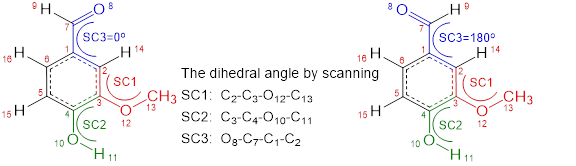Fig. 1. The dihedral angle of molecular structure for vanillin

The dihedral angle rotation potential energy surfaces of aldehyde with methoxyl, and aldehyde with hydroxyl were considered. The potential energy surface of hydroxyl and methoxyl were calculated by the RHF/6-31G and RB3LYP/6-31G methods, respectively, adding redundant coordinates. The initial value of each of the two dihedral angles was -180°, and the step size was 10°, changing from -180° to 180°.

Geometry optimization and energy calculation

All the stable points on the potential energy surface regarded the scanned spatial configuration as the initial values. Full geometry optimization at -B3LYP/6-31G, B3LYP/6-31g(d), B3LYP/6-311G (d, p), MP2/6-311g(d, p), and the frequency calculations were done to obtain the relevant thermodynamic functions for the energy comparison and analysis.

For the energy calculation of optimized global energy minimum conformation, the local energy minimum conformation, and the transition state conformation, the correction coefficients of corresponding calculation methods were used. The frequency correction factor was obtained from the literature (Foresman et al. 1996; Young 2001). The correction factor of B3LYP/6-31G was 0.89; that of B3LYP/6-31g(d) was 0.8953; that of B3LYP/6-311g(d, p) was 0.9051; and that of MP2/6-311g(d, p) was 0.9496. In order to examine the impact of the base group and electron correlation energy on the conformational energy and stability, in the use of MP2/6-311g(d, p) basis set for optimization, we adopted the maximum polarization basis sets including diffuse functions, such as 6-311++g(d, p) basis set.

Hydrogen bonds and bond strength calculation

The G2 combination method was adopted for optimization and the theoretical method of HF, DFT, and MP2 with basis sets of 6-31g(d), 6-311G (d, p), and HF/6-31++g (d, p) were adopted for bond strength calculation.

RESULTS AND DISCUSSION

Potential Energy Surface Scan

Assuming the hydroxyl dihedral angle SC2 as 180°, scanning the methoxyl dihedral SC1 and aldehyde dihedral SC3, the potential energy surface results were obtained, with the results shown in Fig.2. Assuming the methoxyl dihedral SC1 as 0°, scanning the hydroxyl dihedral SC2 and aldehyde dihedral SC3, the potential energy surface results were shown in Fig.3.

As shown in Fig. 2, when the methoxyl dihedral SC1 was 0° and the aldehyde dihedral SC3 was 180°, the energy was predicted to be the lowest. Figure 3 shows when the hydroxyl dihedral SC2 was 0° and the aldehyde dihedral SC3 was 0°, the energy was the lowest.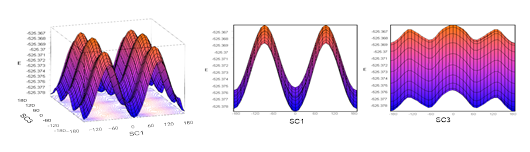Fig. 2.  The PES of vanillin molecule (SC2=180°, SC1/SC3 as variable)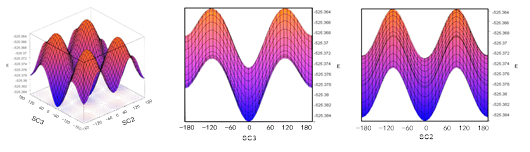Fig. 3.  The PES of vanillin molecule (SC1=0°, SC2/SC3 as variable)

From the above results, the aldehyde dihedral SC3 value related to the methoxy dihedral SC1 value and the hydroxyl dihedral SC2 value. Based on analysis from steric hindrance, it may be that the methoxyl dihedral SC1 in stable conformation tended to 0° to avoid the steric hindrance by the hydroxyl rotating. In this case, 180° for the aldehyde dihedral SC3 was more stable. Thus the following discussion focused on the influence of the changes of methoxy dihedral and hydroxyl dihedral on the energy system when the aldehyde dihedral SC3 was 180°.

Assume the aldehyde dihedral SC3 as 180°, the methoxyl dihedral SC1 and hydroxyl dihedral SC2 were scanned to get the potential energy surface results as shown in Fig. 4.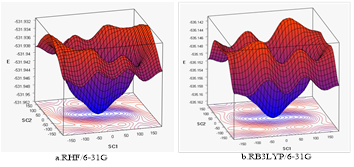Fig. 4.  The PES of vanillin molecule (SC3=180°, SC1/SC2 as variable)

In the structure of the vanillin molecule, the 3 and 4 positions of the benzene ring were connected to a hydroxyl group and a methoxy group, respectively, and they may rotate around the bond axis, so there may be two changeable dihedral angles. When they rotated around the two dihedrals, there would be different conformational changes and different energy. To find out the lowest energy conformation in the global conformation and the local conformation, and various transition state conformations, it is necessary to add a redundancy coordinate to the Gaussian input file to specify the scan range and step size. In the actual calculation, the first dihedral (methoxyl group, 2-3-12-13) was fixed first, and then the second dihedral (hydroxyl, 3-4-10-11) was rotated with step size of 10°. After that, the second dihedral (3-4-10-11) was fixed, and then the first dihedral (2-3-12-13) was rotated, and calculation of rotational energy was made after each rotating. The methods of RHF/6-31G and R3LYP/6-31G yielded the same potential energy surface of vanillin as shown in Fig. 2. The potential energy surface was constituted by 1369 points. The two methods obtained almost same potential energy surface, but the latter (Fig. 3) was calculated by a DFT method, which had higher accuracy and faster speed.

Conformation Analysis

The energy changes of the different spatial orientations of hydroxyl and methoxyl can be observed on the potential energy surface. Figure 5 shows visually the corresponding position of these states or conformation on the potential energy surface. There are four minimum energy state on the potential energy surface, which includes a global minimum energy conformation C1 and the lowest energy conformation of three local (C2, C3, C4). Meanwhile, there are six transition state (TS1- TS6).Fig. 5.  The stationary point in PES of vanillin molecule

Because of the low accuracy of the potential energy surface scanning method and the step of 10°, the potential energy curves could give only rough results to locate a variety of energy state stable points. But it could determine which points were useful for higher accuracy calculations. The initial positions of various steady-state atoms were used as the initial state conformation to optimize fully at a higher level, and then the four lowest energy conformations and six transition state conformation details were obtained.

Figure 6 shows the geometry optimization results of the four lowest energy conformation on the potential energy surface by B3LYP/6-311G(d, p). In the four lowest energy conformation, C1 is the cis conformation, and hydroxyl and methoxyl have the same orientation. The dihedral angle of methoxyl (C2-C3-C12-C13) is 0°, and the dihedral angle of hydroxyl (C2-C3-C12-C13) is 0°. C2 is the trans conformation, and orientation of hydroxyl and methoxyl are opposite. In the conformation, the dihedral angle of methoxyl (C2-C3-C12-C13) is 0°, and the dihedral angle of hydroxyl (C2-C3-C12-C13) is 180°. Since the hydroxyl and the methoxyl have the same orientation in C1 conformation, it is conducive to form hydrogen bonding between the hydrogen atoms on the phenolic hydroxyl and the oxygen atoms on the methoxyl groups. From the point of the energy, this conformation has higher stability than C2, which has the dihedral angle conformation C3-C4-O10-H11 of 180° with reverse arrangement.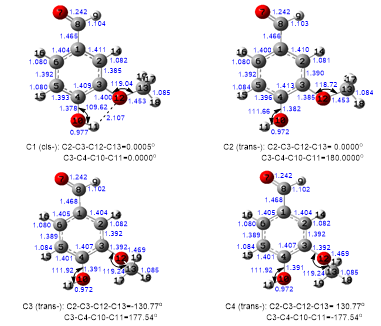Fig. 6.  The four energy minimum conformers

The preliminary results of the potential energy surface scanning of C3: the dihedral angle of methoxyl (C2-C3-C12-C13) is -130° and the dihedral angle of hydroxyl (C2-C3-C12-C13) is 180°; the angle are -130.77° and 177.54° after further optimization. The preliminary results of the potential energy surface scanning of C4 are the dihedral angle of methoxyl (C2-C3-C12-C13) is 130°, and dihedral angle of hydroxyl (C2-C3-C12-C13) is 180°. The values are 130.77° and -177.54° after further optimization. In the two energy conformation, the dihedral angle of hydroxyl approximately maintains trans orientation of C2 conformation (180°), slightly deviating from the benzene plane, while the methoxyl is on the phenyl ring plane up and down, which also has a certain stability.

There were great differences between the spatial orientation of C3 and C4 in vanillin and those in guaiacol calculated by Agache and Popa (2006). The rotating dihedral angle of methoxyl in vanillin was 130.77°, but that was 112.17° in guaiacol, which may be due to the presence of aldehyde in vanillin. This could illustrate the presence of aldehyde influences the stable structure of methoxyl during rotation. From the energy point of view, the conformational energy of C3 or C4 was lower than the conformational energy of C2, which may be due to the methoxyl was not in the plane of the benzene ring to avoid the steric hindrance of the hydroxyl.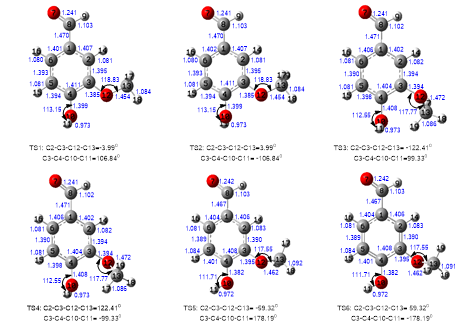Fig. 7.  The six transition state conformers

Figure 7 shows the optimization results of the transition state conformation of the six molecules. In the six transition state conformations (TS1 through TS6), the dihedral angle of the methoxyl (C2-C3-C12-C13) in TS1 is -3.99°, and the dihedral angle of hydroxyl (C2-C3-C12-C13) is 106.8°. The dihedral angle of the methoxyl (C2-C3-C12-C13) in TS2 is 3.99°, and the dihedral angle of the hydroxyl (C2-C3-C12-C13) is -106.84°. In the two conformations, the methoxyl is approximate to maintain the orientation of C1, and the hydroxyl is on the phenyl ring plane up and down. The dihedral angle of the methoxyl (C2-C3-C12-C13) in TS3 is -122.41°, and the dihedral angle of the hydroxyl (C2-C3-C12-C13) is 99.33°. The dihedral angle of methoxyl (C2-C3-C12-C13) in TS4 is 122.41°, and the dihedral angle of the hydroxyl (C2-C3-C12-C13) is -99.33°. Both the hydroxyl and the methoxyl are deviated from the benzene ring plane at a certain angle. The dihedral angle of the methoxyl (C2-C3-C12-C13) in TS5 and TS6 are 59.32°, and the dihedral angle of the hydroxyl (C2-C3-C12-C13) in TS5 and TS6 are -178.19°. In TS5 and TS6, the methoxyl is in the benzene ring plane, while the hydroxyl is on the phenyl ring plane up and down, which is different from the calculation results of guaiacol by Agache and Popa (2006), who only found a transition state of TS5 on the potential energy surface of guaiacol, and the two dihedral angle were both about 180°.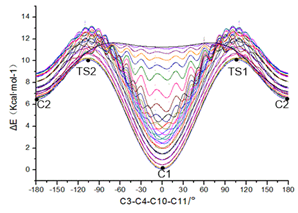Fig. 8.  Change of potential difference with dihedral angle of rotating hydroxyl and methoxyl

Figure 8 shows C1 (0°) the global minimum energy state, and C2 (180°) the local minimum energy state. The interconversion between C1 and C2 is achieved by the symmetrical transition state TS1/TS2.Fig. 9.  Change of potential difference with dihedral angle of methoxyl

Figure 9 shows the C3 and C4 correspond to the two other local energy minimum conformations. The interconversion between C3 and C4 is achieved by the symmetrical transition state TS5/TS6. The conversion from C1 to C3 or C4 must go through the transition state TS1/TS2 first to C2, and then go through the transition state TS5/TS6 into C3 or C4. C1 can also can convert via TS3 to C3, and then go through TS4 to C4, but it must climb a higher energy barrier. When the hydroxyl takes a cis conformation (C3-C4-O10-H11=0°), it is bound to the total energy of the transition state energy close to 12 kcal/mol to make the full rotation of the methoxy. Because when the C2-C3-O12-C13 is approximately to 180, the strong steric hindrance between hydroxyl and methoxyl would be to make it difficult to cross the transition state. When the hydroxyl takes a trans conformation (C3-C4-O10-H11=180°), because the molecular structure is spacious, the methoxyl can take three different spatial orientations. The first conformation corresponds to the C2 (C2-C3-O12-C13=180°), the second one corresponds to the conformational C3 (C2-C3-O12-C13 =130.77°), and the third conformation corresponds to C4 (C2-C3- O12-C13 =-130.77°). C3 and C4 are arranged symmetrically on the potential energy curve, which is non-planar to methoxyl. The conversion from the planar conformation C2 to the non-planar conformation C3 and C4 must be achieved through two transition states TS3 and TS4. The conversion from C3 to C4 can be achieved through another saddle point TS5/TS6.

Table. 1. Geometric Parameter and Relatively Energy Difference of Conformers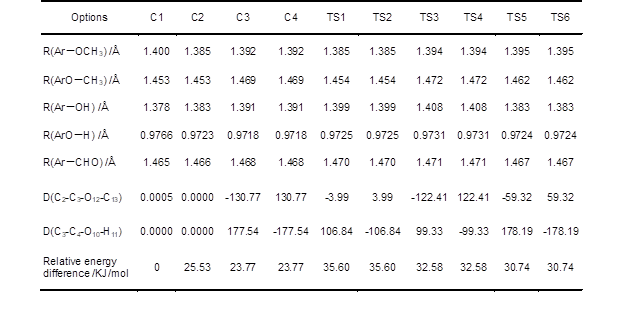The results in Table 1 were calculated on the level of B3LYP/6-311G. Table 1 shows that the minimal bond length between the aromatic ring carbon and methoxyl oxygen (Ar-OCH3) is C2 (1.385Å), and in the other conformations the bond length is 1.392 to 1.400Å. The minimal bond length of ArO-CH3 is C2 (1.453 Å), too. In the conformation of C2, the bond length of Ar-OCH3 and ArO-CH3 is minimal due to its special structure; that is, the hydroxyl is in the trans position, so the steric hindrance between the hydroxyl and the methoxyl is minimal. In the conformation of C1 and C2, phenolic hydroxyl oxygen, and methoxyl oxygen and aryl carbon atoms are all limited on the plane, and the differences of the other bond length in them are very small. The smallest bond length of Ar-OH is C1, which is due to the presence of hydrogen bonds. For the conformation of C3 and C4, the phenol oxygen and methoxyl oxygen are both on the plane of the aromatic ring, and only the methoxyl carbon is out of the plane. In addition to the dihedral angle, the lowest energy (bond lengths and angles) of the four kinds of geometrical parameters have little differences.

From the relative energy difference of each conformation to C1 in Table 1, the lowest energy in all conformations is C1. The energy of planar conformation C2 (25.53 KJ/mol) is higher than the non-planar conformation C3/C4 energy (23.77 KJ/mol). In the six kinds of transition states, the energy of TS5/TS6 (30.74 KJ/mol) is lower than TS3/TS4 (32.58 KJ/mol) and TS1/TS2 (35.60 KJ/mol).

Minimum Energy

The results of the stable conformations of these energy systems and spatial configuration to the global minimum energy conformation (C1) are shown in Table.2. The results were obtained without any restriction molecule conditions, and their full optimizations were in a more advanced level with frequency calculations.

Table. 2. Geometric Parameters of Conformers Calculated by Different Methods for C1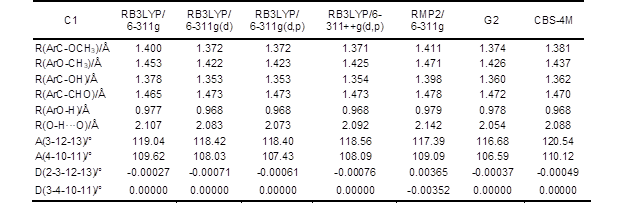Table 2 shows that the geometric parameters results of C1 obtained by different calculation methods have some differences. When different basis sets of B3LYP are adopted, the results are quite different whether or not polarization functions and diffuse functions are adopted. But there is little difference resulting when using the three kinds of basis set of 6-311g(d), 6-311g(d, p) and 6-311++g (d, p). The experimental values of acetyl ketone, phenol, obtained from the literature are shown in Fig. 10.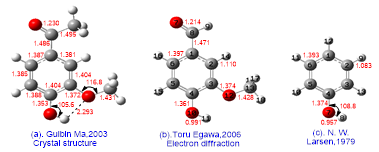Fig. 10. The literature value of structure parameters of model molecules

The experimental results of the model compounds of acetyl vanillin ketone and phenol calculated by higher precision of the combination of methods G2 and CBS-4M are close to the literature values (Larsen 1979) in Fig. 10. For instance, the bond length of Ar-OCH3 obtained by the method of G2 is 1.374 Å, while the literature values is 1.372 Å. The bond length of O-H obtained by MP2/6-311G(d, p) is 0.966Å. Using acetyl vanillin ketone and phenol as references, the bond length of O-H in acetyl vanillin ketone is 0.819Å, and that in phenol is 0.957Å, which accord with literature values (Albinsson et al. 1999; Li et al. 1999). The calculation results by high precision combination method (G2 and CBS-4M) have good consistency with the experimental values.

But there are also parts of the calculation results that are inconsistent with literature values (Elerman et al. 1999), such as the bond length of Ar–OH, whose calculation results by MP2/6-311-g(d, p) is 1.361 Å, that by G2 is 1.360 Å, and that by CBS–4M is 1.362Å, while the literature data is 1.374Å in phenol, and 1.353 Å in acetyl vanillin ketone molecules. The fact that the theoretical and experimental values do not match exactly is also reflected in the calculation of the bond angle, such as C-O-H bond angle, whose calculated value is 106.59°, and that in acetyl vanillin ketones and phenol is 105.62° and 108.77°, while the literature data are 109.62° to 120.52° (Li  and Su 1995).

Transition State

If there is only one imaginary frequency, the state is bound to the transition state conformation. Table 1 shows that the six kinds of transition states are well optimized and the frequency calculations show they all have only one imaginary frequency, indicating that six states are transition states.

From the observation of the potential energy surface, the methoxyl dihedral angle (C2-C3-O12-C13) and the hydroxyl dihedral angle (C3-C4-O10-C11) are close to 180°, so there should be a transition state. But in the transformation of various initial values after looking in the transition state it was not possible to obtain convergence, which is the same condition with guaiacol transition optimized by Agache. The frequency calculation by MP2/6-311g (d, p) indicates three imaginary frequencies: 378.35 icm-1, 241.04 icm-1, and 164.11 icm-1, respectively corresponding to the rotation of hydroxyl, methoxyl, and aldehyde.

The Relative Stability of the Conformation

Three different methods (HF method, DFT, and MP2) and different basis sets for molecular optimization calculation were used with single-point energy calculations, then different conformational energy eigenvalues were obtained. The energy relative to the lowest energy conformations C1 are listed in Table 3.

Table 3 shows that, using the same method with different base groups, the higher complexity in calculations can lead to lower energy difference. Heavy atoms contained d polarization functions and diffuse functions 6-311++G basis set, so that the total correlation can be reduced more. The energy of C2 is 21.00 KJ/mol (HF), 19.59 kJ/mol (B3LYP), and 19.53 kJ/mol (MP2). The energy of C3/C4 value is 17.17 KJ/mol (HF), 24.90 KJ/mol (B3LYP), and 23.30 KJ/mol (MP2). Using the 6-31g(d) basis set with the use of 6-311g(d,p) basis set for molecular geometry optimization results comparison shows that the choice of the basis set on the relative energy difference has little effect (1.43-7.72 KJ/mol). With 6-31g(d) basis set structure optimized by energy difference of 1.51-7.59 KJ/mol (HF), using the 6-311g(d, p) basis set structure optimized by the energy difference 1.43 – 6.43 KJ/mol (MP2) .

Table. 3. Relatively Energy Difference of Conformers (HF, DFT, MP2)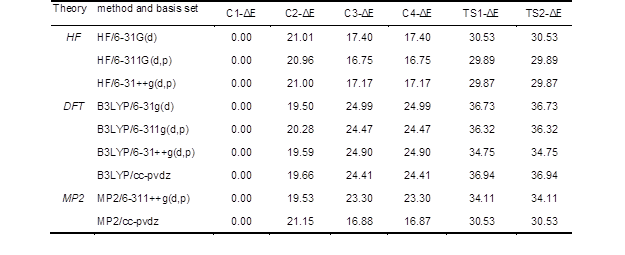Note: Single-point energy calculations are all on the basis of the optimized results by MP2/6-311g(d, p) and the data has been corrected.

Table 3 shows the relative stability of trans conformation related to the calculated methods selected. The results obtained by the method of HF show that non-planar conformations (C3, C4) have greater stability than planar conformation C2, but the results obtained by DFT and MP2 are opposite.

The data above show that the global minimum energy state corresponds to C1, and the group take cis arrangement, because the energy of trans conformation is 19.53 – 39.58 kJ/mol, which is less stable than the cis. In addition, the paired C3/C4 conformation (and TS1/TS2, TS3/TS4, TS5/TS6) energy is basically the same. In fact, in addition to these structural orientations is different, it is very difficult to distinguish from the point of energy.

Hydrogen Bonds

Due to its special structure (a hydroxyl and a methoxy adopting cis- orientation) in C1, there must be an intramolecular hydrogen bond, which was the root causes of its higher stability.

Bond length

The bond length geometry optimized by the combination methods of G2 was 2.054 Å, and the distance between oxygen (O-O) was 2.612 Å, while the experimental data was 2.612 Å with a difference of 0.027 Å.

Hydrogen bond angle

The included angle in hydrogen (O-H-O) was 117.02°, and the hydrogen bond angle was 98.35 – 116.15°. Restricted by geometric parameters changes of two rotation groups, the distance between oxygen (O-O) in p-methoxy phenol compounds was 2.598 – 2.717Å.

Hydrogen bond energy

Hydrogen energy could be understood as the difference between the total energy of open structure (C2) and the total energy of chelate structure (C1). In fact, the differences are relative to the energy of C2 in Table 3. The calculations based on the second level (MP2) and fourth grade (MP4SDQ) Møller-Plesset methods, including diffuse functions polarization 6-311G basis set showed that hydrogen bond energy was 18.09 to 18.51 KJ/mol. Then the hydrogen bond energy calculated by the combination method CBS-4M was 18.52 KJ/mol, which was consistent with the results got by Agache.

Chemical Bond Strength Calculations in Vanillin Molecule

The bond dissociation formula was set up as M (R1-R2) = R1 + R2, and the calculation results were obtained by the following formula. The bond dissociation enthalpy (BDE) is given as follows (Klein and Lukeš 2006),

BDE = H(R1) + H(R2)- H(M)                                                                         (1)

H(X) = E0 + ZPE + ΔHtrans + ΔHrot + ΔHvib + RT                                            (2)

where R 1 and R 2 are free radicals, H(X) is the enthalpy, E0 is the total electron energy, ZPE is the zero-point energy, and ΔHtrans, ΔHrot, and ΔHvib are the contributions of translation, rotation, and vibration, respectively, to the enthalpy.

The fracture mode of vanillin adopted in actual calculations is in Fig. 11.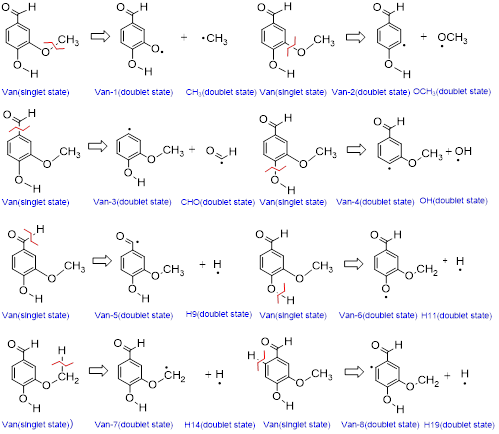Fig. 11. Vanillin molecule cracking to form radical

In the calculation, B3LYP method was adopted on the B3LYP/6-311g(d, p) calculation level, adding d, p polarization functions for optimizing to achieve high accuracy and efficiency. The keywords was “# opt b3lyp/6-311g(d, p) temp = T“, where T was the temperature units of K. The results had been converted into common units of energy (kcal/mol). The correction factor of 6-311G/6-311g(d, p) was 0.9650.

Table. 4. Calculation of Bond Strength on Vanillin at Five Temperatures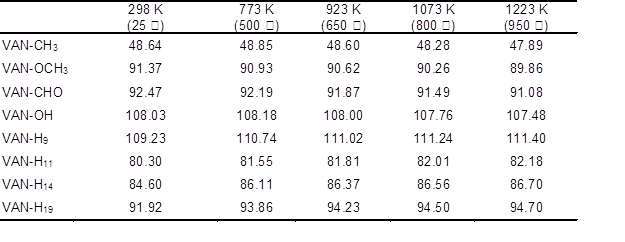Method：b3lyp/6-311g(d, p)

The results show that the temperature has little influence on the bond energy. The bond energy of vanillin molecule (Ar-OCH3, ArO-CH3, Ar-CHO, ArC-OH) decreases slightly as temperature increases, but the condition in the C-H directly connected to the benzene ring, C-H, O-H in aldehyde, and the C-H bond in methyl energy is opposite, which may due to the conjugated structure in benzene ring.

For comparison with literature values, the calculations are achieved on the level of B3LYP/cc-pVQZ as shown in Fig. 12. The results in Fig. 12 are lower than the data by B3LYP/6-311g(d, p) and B3LYP/cc-pVDZ in the literature by Shin et al. (2001). Thus, the different methods and basis sets impact on the calculation results greatly, but in general, each data of bond energy does not change much. As long as the same set of data is used, analysis results are basically the same.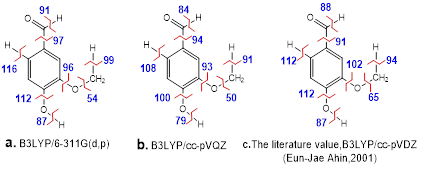Fig. 12. The bond strength of vanillin at 298K

In order to obtain more accurate calculations, the CBS-4M were used for high-precision calculation of bond energy. In the CBS-4M calculation method, the ZPE (correction factor) is 0.91671. The HF/3-21 g(d) is used for geometry optimization, the SCF energy calculation for HF/6-311+G (3d2f, 2df, p), the second correction for MP2/6-31+G, and grade correction for MP4 (SDQ) / 6-31G.

The results obtained by the combination method of CBS are shown in Fig. 13.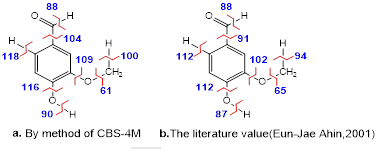Fig. 13. Comparison of bond strength calculation and literature value

The bond energy of ArO-CH3 calculated by CBS-4M is 61 kcal/mol, and the literature value is 65 kcal/mol. Compared to Fig. 12, the data calculated by CBS-4M is closer to the literature value (Shin et al. 2001).

The larger bond strength primarily exists in Ar-OH, Ar-H, Ar-CHO, Ar-OCH3, and C-H not connected on the ring, while the bond strength of ArO-CH3 is particularly small, indicating that the ArO-CH3 will rupture first during thermal cracking. Lignin model compounds have a large number of phenolic pyrolysis products, most likely due to the low strength of the bond to form phenol or catechol compounds.

Ether Bond Strength Calculations in Model Dimer Molecule

The G-type dimers were optimized by MP2/6-31G, and the bond energy, bond length, and other structural parameters of the structure are shown in Fig. 14.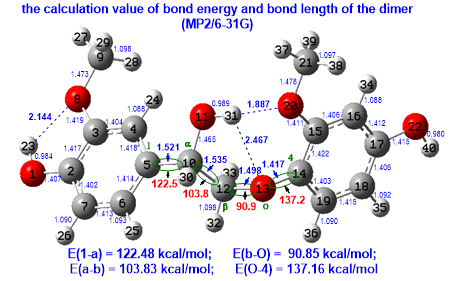Fig. 14. The theoretical calculation results of G-type model dimer

Figure 14 shows that in the dimer 1-α-β-O-4 structure, the stability order of the bond is: O-4> 1-α> α-β> β-O, which is why a large number of phenols retain at the pyrolysis of most biomass or lignin.

CONCLUSIONS

1. The conformational parameters of phenolic hydroxyl and methoxy in guaiacum were fully characterized through the ab initio calculations for vanillin. The hydroxy and methoxy groups could take different spatial orientation. There were four kinds of stable conformations based on hydroxyl, divided into cis- and trans- forms. Depending on whether the methoxyl group was in the plane of the aromatic, there were different trans orientations. After optimization and calculations, there were six saddle points, namely, six transition stat The cis conformation had the minimum total energy.
2. The lowest energy conformation with high stability was due to the intramolecular hydrogen bond. The hydrogen bond energy calculated was 19.53 to 21.15 kcal/mol. The hydrogen bond length was 2.142Å (MP2/6-311G). The larger bond strength primarily existed in Ar-OH, Ar-H, Ar-CHO, Ar-OCH3, and C-H not connected on the ring, while the bond strength of ArO-CH3 was particularly small.
3. In the dimer 1-α-β-O-4 structure, the stability order of the bond was: O-4 > 1-α > α-β > β-O, The O-4 bond is most stable and β-O bond is easier cleavage to produce phenols, which agreed well with the fact that there are a lot of phenolic compounds in pyrolysis products of biomass or lignin.
4. High precision methods include combining methods (including G2 combination method, complete basis set CBS methods, et al.) can be used in further work, and the IRC path analysis can be adopted in molecular cracking to predict the reaction path and activation energies theoretically.

ACKNOWLEDGEMENTS

The authors greatly acknowledge the support of the National Basic Research Program of China (973 program, No.2013CB228101) and the Hubei Collaborative Innovation Center for Rare Metal Chemistry, Hubei Normal University.

REFERENCES CITED

Agache, C., and Popa, V. (2006). “Ab initio studies on the molecular conformation of lignin model compounds. I. Conformational preferences of the phenolic hydroxyl and methoxy groups in guaiacol,” Monatshefte für Chemie 137(1), 55-68.

Albinsson, B., Li, S., Lundquist, K., and Stomberg, R. (1999). “The origin of lignin fluorescence,” Journal of Molecular Structure 508(1-3), 19-27.

Egawa, T., Kameyama, A., and Takeuchi, H. (2006). “Structural determination of vanillin, isovanillin and ethylvanillin by means of gas electron diffraction and theoretical calculations,” Journal of Molecular Structure 794(1-3), 92-102.

Elerman, Y., Elmali, A., Kendi, E., and Özbey, S. (1999) “4-(2,5-Di-tert-butylphenyl nitrilomethylidyne)-2-methoxyphenol,” Acta Crystallographica Section C 55(1), 116-119.

Foresman, J. B., Frisch A. (1996). Exploring Chemistry with Electronic Structure Methods, Gaussian Inc., Wallingford USA Frisch, M. J., Trucks, G. W., Schlegel, H. B., and Pople, J. A. (2004). Gaussian 03, Revision E.01, Gaussian, Inc., Wallingford, CT, USA.

Jakobsons, J., Gravitis, J., and Dashevskii, V. G. (1981). “A theoretical analysis of the conformations of model compounds of lignin. A study of internal rotation, the relative stablity of the rotamers and interamolecular H-bond in the molecules of m-methoxybenzaldehyde and vanillin,” Zhurnal Strukturnoi Khimii 22(2), 179-186.

Klein, E., and Lukeš, V. (2006) “DFT/B3LYP study of O–H bond dissociation enthalpies of para and meta substituted phenols: Correlation with the phenolic C–O bond length,” Journal of Molecular Structure: THEOCHEM 767(1-3), 43-50.

Larsen, N. W. (1979). “Microwave spectra of the six mono-13C-substituted phenols and of some monodeuterated species of phenol. Complete substitution structure and absolute dipole moment,” Journal of Molecular Structure 51(8), 175-178.

Li, S., Lundquist, K., and Stomberg, R. (1999). “An investigation of 2,4-dihydroxy-3,3-dimeth-oxy-5-methylstilbene using X-ray crystallography and NMR spectroscopy,” Acta Crystallographica Section C 55(6), 1012-1014.

Li, Z. D., and Su, G. (1995). “2-Methoxy-5-(4-nitrostyryl) phenol,” Acta Crystallographica Section C 51(2), 311-313.

Ma, G., Patrick, O. B., Hu, Q. T., and James, R. B. (2003). “4-Hydroxy-3-methoxy acetophenone (acetovanillone),” Acta Crystallographica Section E 59(4), 579-580.

Remko, M. (1979). “MO investigations on lignin model compounds VIII. A PCILO study of intramolecular hydrogen bond in guaiacol and o-vanillin,” Advances in Molecular Relaxation and Interaction Processes 14(4), 315-320.

Shin, E. J., Nimlos, M. R., and Evans, R. J. (2001) “A study of the mechanisms of vanillin pyrolysis by mass spectrometry and multivariate analysis,” Fuel 80, 1689-1696.

Tylli, H., Konschin, H., and Grundfelt-Forsius, C. (1981). “A Raman spectroscopic study of the low-frequency vibrations in guaiacol and its deuterated analogues,” Journal of Molecular Structure 77(1-2), 37-50.

Young, D. (2001). Computational Chemistry: A Practical Guide for Applying Techniques to RealWorld Problems, John Wiley & Sons Inc., New York.

Article submitted: November 17, 2014; Peer review completed: Jan. 20, 2015; Revised version received: September 26, 2015; Further revisions: November 11, 2015; Acceptance: November 19, 2015; Published: December 4, 2015.

DOI: 10.15376/biores.11.1.1044-1060